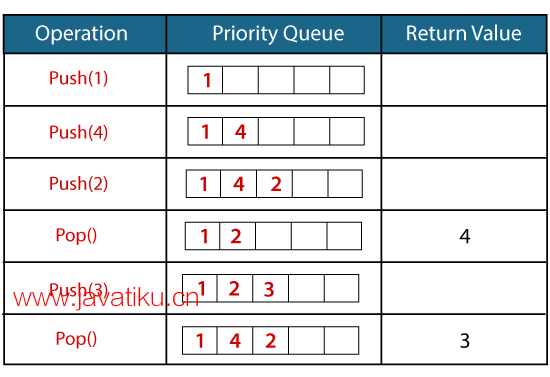# C++教程-C++中的优先队列## C++中的优先队列

C++中的优先队列是STL中的派生容器，它只考虑最高优先级的元素。队列遵循FIFO（先进先出）策略，而优先队列根据优先级弹出元素，即最高优先级的元素首先弹出。

• 在优先队列中，队列中的每个元素都与某个优先级相关联，而队列数据结构中不存在优先级。
• 优先队列中具有最高优先级的元素将首先被删除，而队列遵循先进先出（FIFO）策略，即先插入的元素将首先被删除。
• 如果存在多个具有相同优先级的元素，则将考虑队列中元素的顺序。

### 优先队列的语法

``priority_queue<int> variable_name; ``### 优先队列的成员函数

push()向优先队列中插入新元素。
pop()从队列中删除具有最高优先级的顶部元素。
top()用于访问优先队列的顶部元素。
size()确定优先队列的大小。
empty()验证队列是否为空。根据验证结果返回状态。
swap()将一个优先队列的元素与具有相同类型和大小的另一个队列交换。
emplace()在优先队列的顶部插入新元素。

``````#include <iostream>
#include <queue>
using namespace std; int main()
{
priority_queue<int> p; // 变量声明。
p.push(10); // 在队列中插入10，top=10
p.push(30); // 在队列中插入30，top=30
p.push(20); // 在队列中插入20，top=20
cout << "Number of elements available in 'p': " << p.size() << endl;
while (!p.empty())
{ std::cout << p.top() << std::endl;
p.pop();
}
return 0;
} ``````

``Number of elements available in 'p': 3 30 20 10``

``````#include <iostream>
#include <queue>
using namespace std;
int main()
{
priority_queue<int> p; // 优先队列声明 priority_queue<int> q; // 优先队列声明
p.push(1); // 在p中插入元素'1'。
p.push(2); // 在p中插入元素'2'。
p.push(3); // 在p中插入元素'3'。
p.push(4); // 在p中插入元素'4'。
q.push(5); // 在q中插入元素'5'。
q.push(6); // 在q中插入元素'6'。
q.push(7); // 在q中插入元素'7'。
q.push(8); // 在q中插入元素'8'。
p.swap(q);
std::cout << "Elements of p are: " << std::endl; while (!p.empty())
{
std::cout << p.top() << std::endl;
p.pop();
}
std::cout << "Elements of q are:" << std::endl; while (!q.empty())
{ std::cout << q.top() << std::endl;
q.pop();
}
return 0;
}``````

``Elements of p are: 8 7 6 5 Elements of q are: 4 3 2 1``